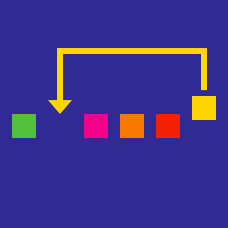Computer Science

# Insertion/Bubble Sorting

What is the best time complexity of bubble sort?

What is the minimum number of swaps operations a standard insertion sort implementation performs on the following list of numbers?

[20, 54, 37, 15, 64, 5, 65, 32, 13, 53, 23, 69, 39, 1, 24, 60, 33, 58, 63]

An inversion is the pair of elements $(i,j)$ in $A[0......n]$ such that $i and $A[i]>A[j]$.

Given an array of $n$ distinct elements, what would be the worst time complexity of an insertion sort algorithm if the array has at most $n$ inversions?

×